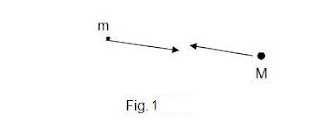## Tuesday, May 28, 2013

### Newton's Law of Gravity

Newton’s law of gravityFig 1

(1) F = –(GmM)/R2

In vector form, this reads as:

(2) F = – [(GmM)/R2]er

Consider a system of particles. We label the particles 1, 2, 3... i...j...Fig 2

(3)F = – ∑i≠j[(GmiMj)/Rij2]er

Define Gravitational Field:

(4) A(x) = F(x)/mi

Substituting (3),

(5) A = = – ∑i≠j[(GMj)/Rij2]er

Gauss Theorem

(6) ∫v A dV = ∫s A•dS

Substitute (5) into the right hand side of (6),

(7) ∫s A•dS = – ∫s[(GM)/R2]er•dS

However, the magnitude of the infinitesimal area element dA is just the area of the infinitesimal solid angle dΩ, given by,

(8) dS = R2er

Substitute (8) into (7),

(9) ∫s A•dS = – ∫s[(GM)/R2]er•R2er

= – GM ∫serer

= – GM ∫sdΩ ,(er2=1)

Therefore,

(10) ∫s A•dS = –4πGM

But the mass can be written in terms of the density,

(11) M = ∫v ρdV

Therefore equation (10) becomes,

(12) ∫s A•dS = –4πG∫v ρdV

Using Gauss Law , equation (6),

(13) ∫s A•dS = ∫v A dV = –4πG∫v ρdV

Therefore,

(14) ∇•A = – 4πGρ

Define the Gravitational Potential:

(15) A = –∇φ

We get,

(16) ∇2φ = 4πGρ

Note that Gauss theorm allows us to say that a particle is attracted as if all the other matter was concentrated at the center of a sphere.